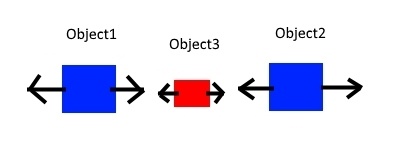# How do i center an object

Hi!
If there 3 object. How i but object3 to the center with two another object. Object1 and object2 move left and right and distance of these two object is changing. But object3 must be centre between them and move too. Object1 and object2 move across too. Hopefully somebody understand what i mean and can help and if there example too. Thanks!To center in X position it should be something like:

``Do = (Object1.X() + Object2.X())/2 to the X position of Object3``

As you can see I’m just calculating the average between the two objectsNow it will work fine if the Origin point of every object is at the center, by default it’s false, the origin point is at the top-left of each object, using the center point for Object1 and Object2 instead:

``Do = (Object1.PointX(Centre) + Object2.PointX(Centre))/2 to the X position of Object3``

And if the Origin point of Object3 is at the top-left and you want Object3 to be fully centered:

``Do = (Object1.PointX(Centre) + Object2.PointX(Centre))/2 - Object3.Width()/2 to the X position of Object3``

The same for Y:

``Do = (Object1.PointY(Centre) + Object2.PointY(Centre))/2 - Object3.Height()/2 to the Y position of Object3``

Let me know if it works, as I haven’t tried it in GD1 Like

Thank you fast answer! I try do some camera test also with that and I think i can do it now. I Let you know if it works.

works perfectly. Thanks again!